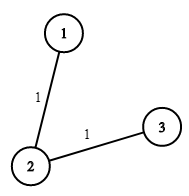# 1168. Optimize Water Distribution in a Village

Optimize Water Distribution in a Village - LeetCode There are n houses in a village. We want to supply water for all the houses by building wells and laying pipes. For each house i, we can either build a well inside it directly with cost wells[i - 1] (note the -1 due to 0-indexing), or pipe in water from another well to it. The costs to lay pipes between houses are given by the array pipes, where each pipes[j] = [house1j, house2j, costj] represents the cost to connect house1j and house2j together using a pipe. Connections are bidirectional. Return the minimum total cost to supply water to all houses.

Example 1:Input: n = 3, wells = [1,2,2], pipes = [[1,2,1],[2,3,1]] Output: 3 Explanation: The image shows the costs of connecting houses using pipes. The best strategy is to build a well in the first house with cost 1 and connect the other houses to it with cost 2 so the total cost is 3.

• code
``````class Solution:
def minCostToSupplyWater(self, n: int, wells: List[int], pipes: List[List[int]]) -> int:

root = [i for i in range(n+1)]
self.cost = 0

def find(x):
if x != root[x]:
root[x] = find(root[x])
return root[x]

def union(x, y, cost):
rtx = find(x)
rty = find(y)
if rtx == rty:
return
root[rtx] = rty
self.cost += cost

# virtual node 0, the cost to each node is the cost of that well, so we only consider edges
for i, well in enumerate(wells):
pipes.append([0, i+1, well])

pipes.sort(key = lambda x: x)

for x, y, cost in pipes:
union(x, y, cost)

return self.cost
``````